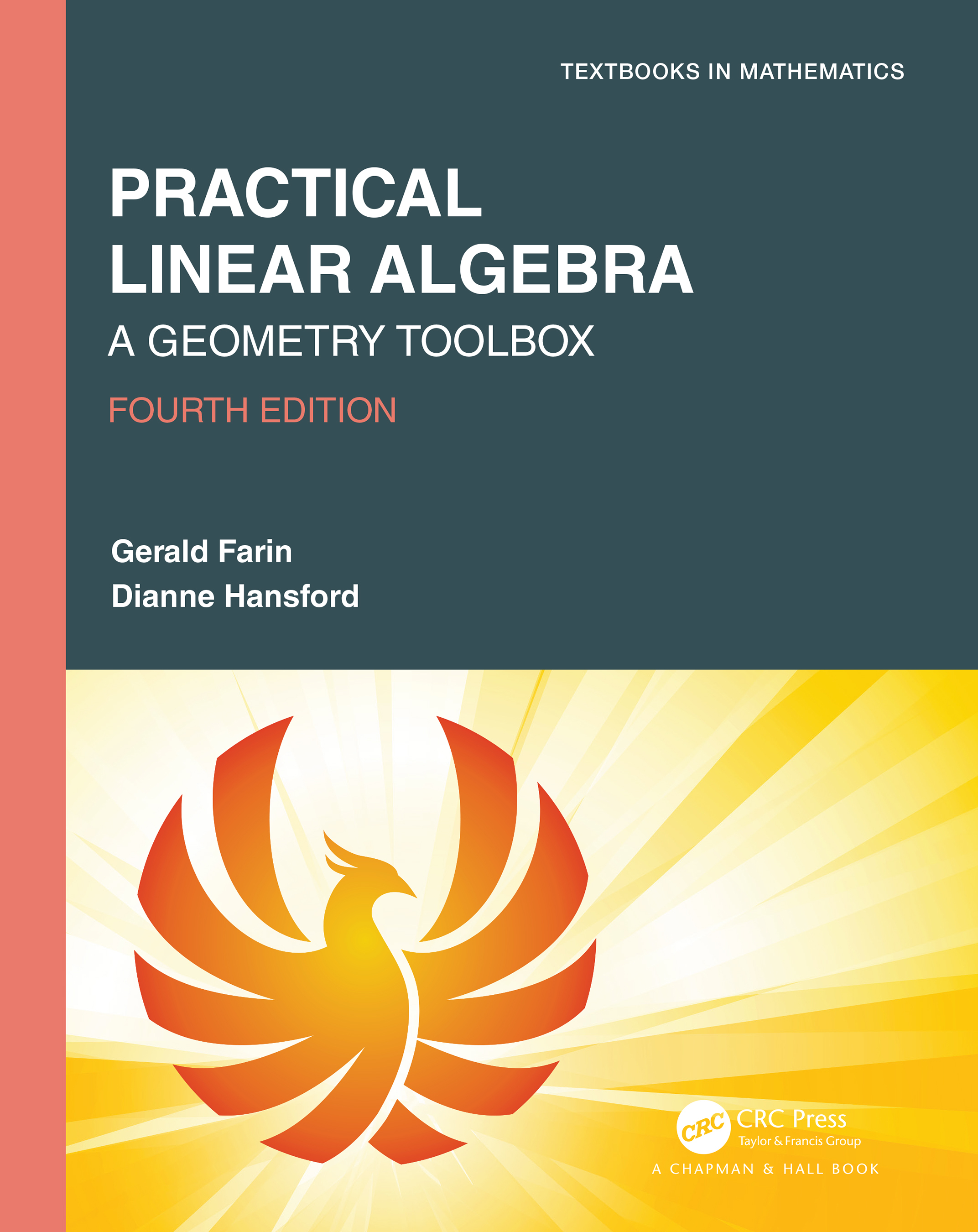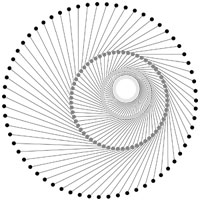Practical Linear Algebra: A Geometry Toolbox, Fourth Edition Gerald Farin & Dianne Hansford A K Peters/CRC Press 2021 592 Pages, 319 B/W Illustrations ISBN: 9780367507848

home

Linear algebra is growing in importance. 3D entertainment, animations in movies and video games are developed using linear algebra. Animated characters are generated using equations straight out of this book. Linear algebra is used to extract knowledge from the massive amounts of data generated from modern technology.

The Fourth Edition of this popular text introduces linear algebra in a comprehensive, geometric, and algorithmic way. The authors start with the fundamentals in 2D and 3D, then move on to higher dimensions, expanding on the fundamentals and introducing new topics, which are necessary for many real-life applications and the development of abstract thought. Applications are introduced to motivate topics.

The subtitle, A Geometry Toolbox, hints at the book’s geometric approach, which is supported by many sketches and figures. Furthermore, the book covers applications of triangles, polygons, conics, and curves. Examples demonstrate each topic in action.

Designed for a one-semester linear algebra course at the undergraduate level, this book gives instructors the option of tailoring the course for the primary interests: math, engineering, science, computer graphics, and geometric modeling.

This practical approach to a linear algebra course, whether through classroom instruction or self-study, is unique to this book.

Instead of using the standard theorem-proof approach, the text presents many examples and instructional illustrations to help students develop a robust, intuitive understanding of the underlying concepts.

#### Features

• More than 120 numerical examples
• 319 illustrations
• WYSK (What You Should Know) summary of main points at the end of each chapter
• Over 200 exercises at the end of each chapter with selected solutions in an appendix
• Extensive glossary
• Robust index
• An appendix with a list of applications that appear in the text
• For instructors: solutions manual, lecture slides, and test bank are available

#### New to the Fourth Edition

• Ten new application sections.
• A new section on change of basis. This concept now appears in several places
• Chapters 14-16 on higher dimensions are notably revised
• A deeper look at polynomials in the gallery of spaces.
• Introduces the QR decomposition and its relevance to least squares.
• Similarity and diagonalization are given more attention as are eigenfunctions.
• A longer thread on least squares, running from orthogonal projections to a solution via SVD and the pseudoinverse.
• More applications for PCA have been added.
• More examples, exercises, and more on the kernel and general linear spaces.

 Preface Descartes’ Discovery Here and There: Points and Vectors in 2D Lining Up: 2D Lines Changing Shapes: Linear Maps in 2D 2 × 2 Linear Systems Moving Things Around: Aﬃne Maps in 2D Eigen Things 3D Geometry Linear Maps in 3D Aﬃne Maps in 3D Interactions in 3D Gauss or Linear Systems Alternative System Solvers General Linear Spaces Eigen Things Revisited The Singular Value Decomposition Breaking It Up: Triangles Putting Lines Together: Polylines and Polygons Conics Curves Appendices A. Applications B. Glossary C. Select Exercise SolutionsWhat's different about our approach to Linear Algebra?

The figures are not included as window dressing, in fact they play an important role in bringing the reader to a robust understanding of the mathematics. However they are not only instructional, they are also fun!Our motivation for writing PLA is described in our Fall 2005 TIES Magazine (design and technology education) article, "A Practical Approach to Teaching Linear Algebra."